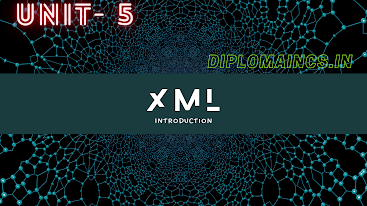## 5/14/20

### Digital Electronics and microprocessor 2019 question

Sub Code: - 1618402

2019(EVEN)

Time: 3Hrs

Semester  IV(New)

D E M

Full Marks: 70

Pass marks: 28

Group A

Choose the most suitable answer from the following options: -   (1*20=20)

(i) Which of the following is minimum error code?

(a) Octal code

(b) Gray code

(c) Binary code

(d) Excess 3-code

(ii) A NOT gate has-

(a) Two input and one output

(b) One input and one output

(c) One input and two output

(d) None of these

(iii) A set of instruction written in computer language is called the

(a) Software

(b) Hardware

(c) Program

(d) None of these

(iv) 1’s complement of 10101 is given by

(a)01010

(b) 01011

(c) 10101

(d)  00100

(v) The shift register is designed to shift data

(a) Only to left changing the order of the nits

(b) Only to right changing the order of the bits

(c) Either left or right changing the order of the bits

(d) Either left or right without changing the order of the bits

(vi) The 8085 instruction RAL performs the following operations

(a) Rotate the content of accumulator to the right

(b)  Rotate the content of accumulator to the left

(c) Rotate the content of accumulator to the right through carry

(d) Rotate the content of accumulator to the left through carry

(vii) Disc and Drum are the

(a) Hardware part of the computer

(b) Software part

(c) Firmware

(d) None of these

(viii) Which of the following memories normally has highest storage capacity?

(a) Magnetic disk

(b) Magnetic tape

(c) Semi conductor memory

(d) Core memory

(ix) OP-AMP’s can be used to amplify:

(a) D.C. input signal

(b) A.C. input signal

(c) D.C. as well as A.C. input signal

(d) None of these

(x)  Number of interrupts of Intel 8085 are

(a) 5

(b) 3

(c) 4

(d) 2

(xi)A program written in mnemonics are called

(a) High level language program

(b) Low level language program

(c) Assembly level language program

(d) None of these

(xii) One of the method is used for A/D conversion is:

(b) Weighted binary resistors

(c) Successive approximation

(d) None of these

(xiii) In a microcomputer _________ is its heart

(a) Microprocessor

(b) ALU

(c) Control Unit

(d) None of these

(xiv) An R-S flip flop cannot be used to design T-type flip flop as

(a) R=0, S=1 is not permitted

(b) R=0, S=0 is not permitted

(c) R=1, S=0 is not permitted

(d) R=1, S=1 is not permitted

(xvi) Flip flop comes under the category of :

(a) 2- bit storage device

(b) 1- bit storage device

(c) Multi bit storage device

(d) None of these

(xvii) Extremely low power dissipation and low cost can be achieved in:

(a) MOS ICs

(b)  CMOS ICs

(c) TTL ICs

(d) ECL ICs

(xviii) The 8085 instruction INR r comes in the category of

(a) Machine control group

(b) Branch group

(c) Arithmetic group

(d) Data transfer group

(xix) An idea OP-AMP has

(a) Infinite Av

(b) Infinite Ri

(c) Zero

(d) All of the above

Group:-"B"

Answer all Five Questions: -                         (5*4=20)

2. Write down the characteristics of an ideal op-amp.

OR

Design Ex-OR gate using only NAND gates.

3. Explain the following two forms of expressing any logic function

I.          Sum of products (SOP) form

II.          Product of sums (POS) form

OR

Draw the logic circuit represented by using the following Boolean expression

i.                Z = AB + CD

ii.              Z = AB + C

4. Discuss the function of ALU of 8085.

OR

Explain the ring counter with a block diagram.

6. What are the functions of an accumulator? Describe

OR

Write a short introduction about Intel 8086 microprocessor.

Group:- "C"

Answer all Five Questions: -                         (5*6=30)

7. Describe the operation of

i.          Decoder and

ii.          Encoder with block diagram

OR

Minimize the following using K-map

f (a, b, c, d) = Σ m (0, 1, 3, 4, 7, 9, 10, 14, 15)

8. Draw the schematic diagram for R-S flip flop using NAND gates. Explain its action. For which combination of input the race around condition observed.

OR

What are the main features of the synchronous counter? Explain, also give the main difference between register and counter.

9. Write an assembly language program to add 8 bits numbers. The sum may be of 16 bits.

OR

Draw the pin diagram of 8085 microprocessor. Explain the function of some of the control pins.

10. Draw the logic diagram, truth table and waveform for a two flip flop ripple counter.

OR

Show how to convert S-R flip flop to J-K flip flop.

11. What are the various addressing modes of 8085 microprocessor? Explain.

OR

Write short notes on any two

i.                D/A convertor

ii.              555- timer

iii.           Stack pointer

iv.            Status flags of 8085